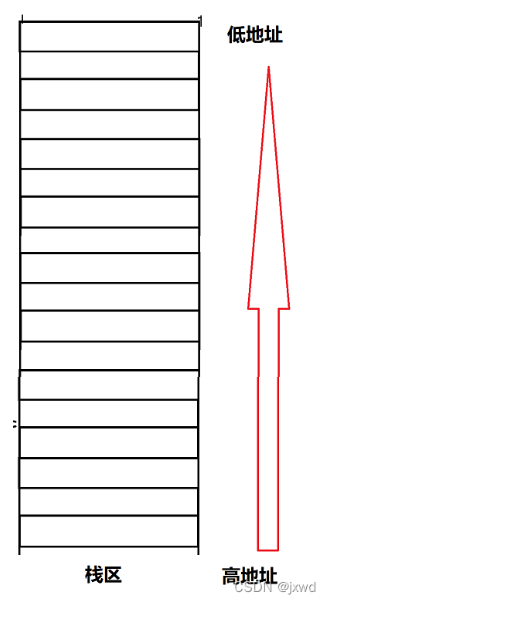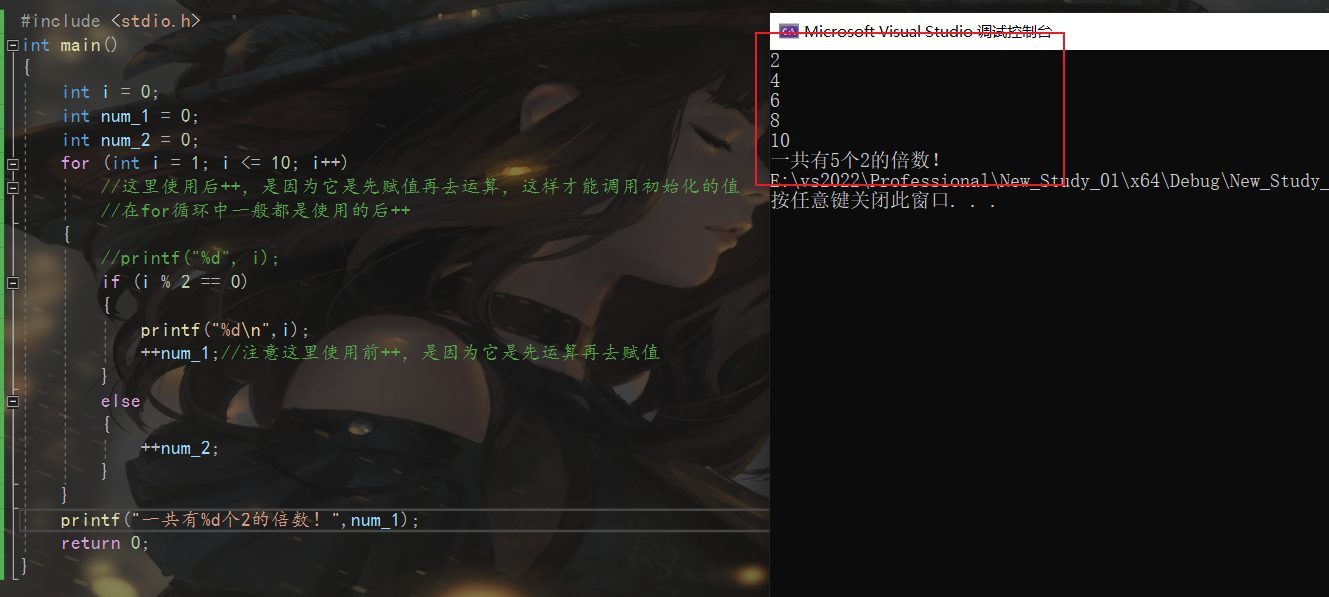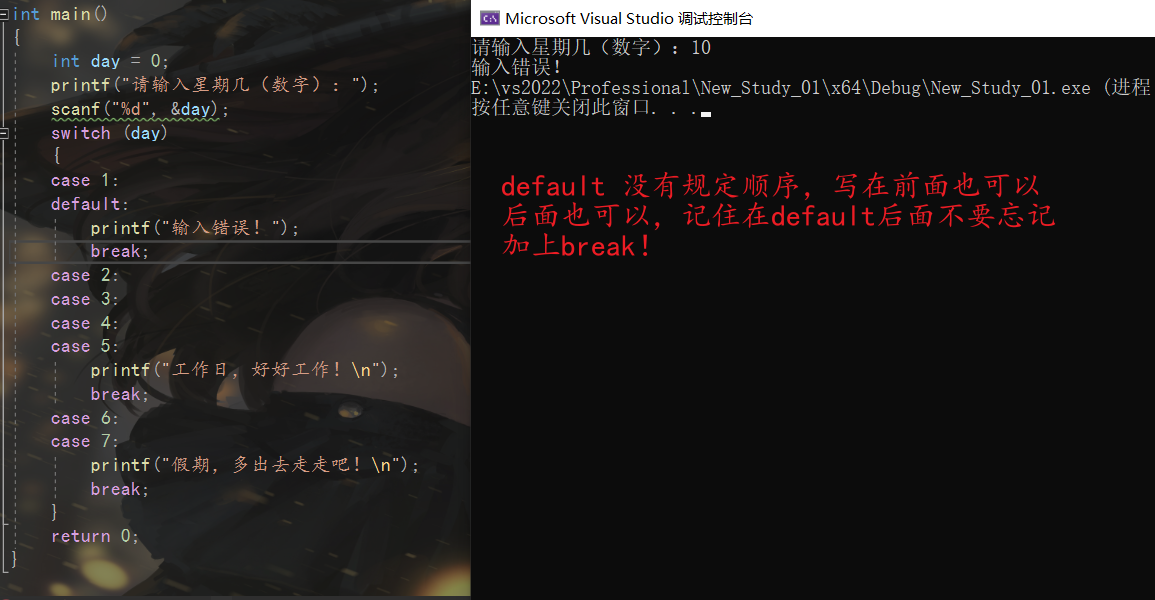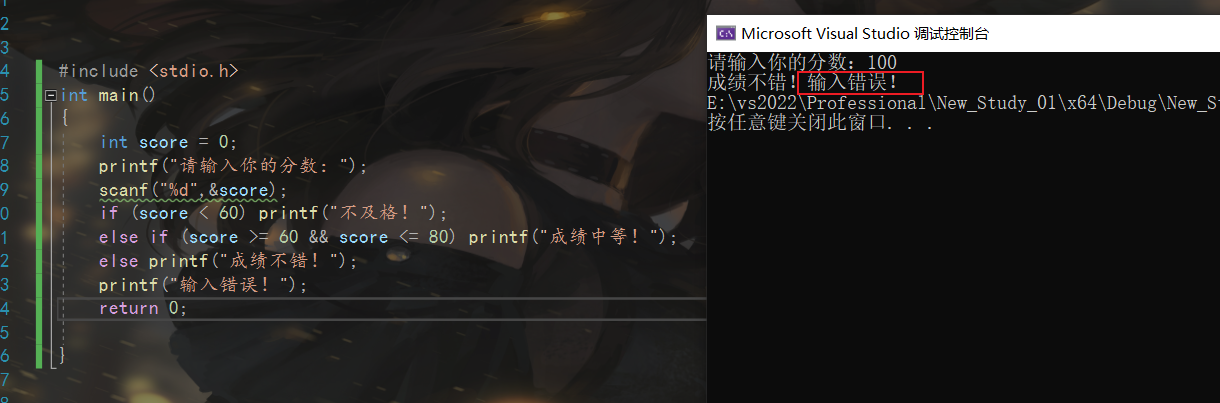# C语言ASM汇编内嵌语法【转】

+关注继续查看
GCC 支持在C/C++代码中嵌入汇编代码，这些汇编代码被称作GCC Inline ASM——GCC内联汇编。这是一个非常有用的功能，有利于我们将一些C/C++语法无法表达的指令直接潜入C/C++代码中，另外也允许我们直接写 C/C++代码中使用汇编编写简洁高效的代码。

1.基本内联汇编

GCC中基本的内联汇编非常易懂，我们先来看两个简单的例子：

__asm__("movl %esp,%eax"); // 看起来很熟悉吧！

__asm__("
movl 1,1,0x80
");

__asm__(
"movl 1,1,0x80" \
);

__asm__ __volatile__("Instruction List");

1、__asm__

__asm__是GCC关键字asm的宏定义：

#define __asm__ asm

__asm__或asm用来声明一个内联汇编表达式，所以任何一个内联汇编表达式都是以它开头的，是必不可少的。

2、Instruction List

Instruction List是汇编指令序列。它可以是空的，比如：__asm__ __volatile__(""); 或__asm__ ("");都是完全合法的内联汇编表达式，只不过这两条语句没有什么意义。但并非所有Instruction List为空的内联汇编表达式都是没有意义的，比如：__asm__ ("":::"memory"); 就非常有意义，它向GCC声明：“我对内存作了改动”，GCC在编译的时候，会将此因素考虑进去。

cat example1.c  int main(int __argc, char* __argv[])  {  int* __p = (int*)__argc;   (*__p) = 9999;   //__asm__("":::"memory");   if((*__p) == 9999)  return 5;   return (*__p);  }  在 这段代码中，那条内联汇编是被注释掉的。在这条内联汇编之前，内存指针__p所指向的内存被赋值为9999，随即在内联汇编之后，一条if语句判断__p 所指向的内存与9999是否相等。很明显，它们是相等的。GCC在优化编译的时候能够很聪明的发现这一点。我们使用下面的命令行对其进行编译：cat example1.c  int main(int __argc, char* __argv[])  {  int* __p = (int*)__argc;   (*__p) = 9999;   //__asm__("":::"memory");   if((*__p) == 9999)  return 5;   return (*__p);  }  在 这段代码中，那条内联汇编是被注释掉的。在这条内联汇编之前，内存指针__p所指向的内存被赋值为9999，随即在内联汇编之后，一条if语句判断__p 所指向的内存与9999是否相等。很明显，它们是相等的。GCC在优化编译的时候能够很聪明的发现这一点。我们使用下面的命令行对其进行编译：gcc -O -S example1.c

catexample1.smain:pushlcatexample1.smain:pushl9999, (%eax) # (*__p) = 9999
movl 5,5, gcc -O -S example1.c

catexample1.smain:pushlcatexample1.smain:pushl9999, (%eax) # (*__p) = 9999
#APP

# __asm__("":::"memory")
#NO_APP
cmpl 9999,(9999,(5, %eax # true, return 5
jmp .L2
.p2align 2
.L3:
movl (%eax), %eax
.L2:
popl %ebp
ret

__asm__("movl %eax, %ebx
sti
popl %edi
subl %ecx, %ebx");

__asm__("movl %eax, %ebx; sti
popl %edi; subl %ecx, %ebx");

__asm__("movl %eax, %ebx; sti\n\t popl %edi
subl %ecx, %ebx");

__asm__("movl %eax, %ebx
sti\n"
"popl %edi;"
"subl %ecx, %ebx");

__asm__("movl %eax, %ebx; sti\n\t"
"popl %edi; subl %ecx, %ebx");

__asm__("movl %eax, %ebx; sti\n\t popl %edi\n"
"subl %ecx, %ebx");

__asm__("movl %eax, %ebx; sti\n\t popl %edi;" "subl %ecx, %ebx");

__asm__(".align 2\n\t"
"movl %eax, %ebx\n\t"
"test %ebx, %ecx\n\t"
"jne error\n\t"
"sti\n\t"
"error: popl %edi\n\t"
"subl %ecx, %ebx");

3、__volatile__

__volatile__是GCC关键字volatile的宏定义：

#define __volatile__ volatile

__volatile__ 或volatile是可选的，你可以用它也可以不用它。如果你用了它，则是向GCC声明“不要动我所写的Instruction List，我需要原封不动的保留每一条指令”，否则当你使用了优化选项(-O)进行编译时，GCC将会根据自己的判断决定是否将这个内联汇编表达式中的指 令优化掉。

2、带有C/C++表达式的内联汇编

GCC允许你通过C/C++表达式指定内联汇编中"Instrcuction List"中指令的输入和输出，你甚至可以不关心到底使用哪个寄存器被使用，完全靠GCC来安排和指定。这一点可以让程序员避免去考虑有限的寄存器的使用，也可以提高目标代码的效率。

__asm__ (" " : : : "memory" ); // 前面提到的

__asm__ ("mov %%eax, %%ebx" : "=b"(rv) : "a"(foo) : "eax", "ebx");

__asm__ __volatile__("lidt %0": "=m" (idt_descr));

__asm__("subl %2,%0\n\t"
"sbbl %3,%1"
: "=a" (endlow), "=d" (endhigh)
: "g" (startlow), "g" (starthigh), "0" (endlow), "1" (endhigh));

__asm__　__volatile__("Instruction List" : Output : Input : Clobber/Modify);

1. Output

Output用来指定当前内联汇编语句的输出。我们看一看这个例子：

__asm__("movl %%cr0, %0": "=a" (cr0));

cat example2.c  int main(int __argc, char* __argv[])  {  int cr0 = 5;   __asm__ __volatile__("movl %%cr0, %0":"=a" (cr0));   return 0;  }cat example2.c  int main(int __argc, char* __argv[])  {  int cr0 = 5;   __asm__ __volatile__("movl %%cr0, %0":"=a" (cr0));   return 0;  } gcc -S example2.c

catexample2.smain:pushlcatexample2.smain:pushl4, %esp
movl 5,4(5,−4(0, %eax
leave
ret

cat example3.c  int main(int __argc, char* __argv[])  {  int cr0 = 5;   __asm__ __volatile__("movl %%cr0, %0" : "+a" (cr0));   return 0;  }cat example3.c  int main(int __argc, char* __argv[])  {  int cr0 = 5;   __asm__ __volatile__("movl %%cr0, %0" : "+a" (cr0));   return 0;  } gcc -S example3.c

catexample3.smain:pushlcatexample3.smain:pushl4, %esp
movl 5,4(5,−4(0, %eax
leave
ret

__asm__(
"movl %%eax, %0 \n\t"
"pushl %%ebx \n\t"
"popl %1 \n\t"
"movl %1, %2"
: "+a"(cr0), "=b"(cr1), "=c"(cr2));

2、Input

Input域的内容用来指定当前内联汇编语句的输入。我们看一看这个例子：

__asm__("movl %0, %%db7" : : "a" (cpu->db7));

cat example4.c  int main(int __argc, char* __argv[])  {  int cr0 = 5;   __asm__ __volatile__("movl %0, %%cr0"::"a" (cr0));   return 0;  }cat example4.c  int main(int __argc, char* __argv[])  {  int cr0 = 5;   __asm__ __volatile__("movl %0, %%cr0"::"a" (cr0));   return 0;  } gcc -S example4.c

catexample4.smain:pushlcatexample4.smain:pushl4, %esp
movl 5,4(5,−4(0, %eax
leave
ret

3. Operation Constraint

1、寄存器约束

__asm__ __volatile__("movl %0, %%cr0"::"eax" (cr0));

__asm__ __volatile__("movl %0, %%cr0"::"a" (cr0));

unsigned short __shrt;

__asm__ ("mov %0，%%bx" : : "a"(__shrt));

movw -2(%ebp), %ax # %ax = __shrt
#APP
movl %ax, %bx
#NO_APP

r I,O 表示使用一个通用寄存器，由GCC在%eax/%ax/%al, %ebx/%bx/%bl, %ecx/%cx/%cl, %edx/%dx/%dl中选取一个GCC认为合适的。
q I,O 表示使用一个通用寄存器，和r的意义相同。
a I,O 表示使用%eax / %ax / %al
b I,O 表示使用%ebx / %bx / %bl
c I,O 表示使用%ecx / %cx / %cl
d I,O 表示使用%edx / %dx / %dl
D I,O 表示使用%edi / %di
S I,O 表示使用%esi / %si
f I,O 表示使用浮点寄存器
t I,O 表示使用第一个浮点寄存器
u I,O 表示使用第二个浮点寄存器

2、内存约束

cat example5.c  // 本例中，变量sh被作为一个内存输入  int main(int __argc, char* __argv[])  {  char* sh = (char*)&__argc;   __asm__ __volatile__("lidt %0" : : "m" (sh));   return 0;  }cat example5.c  // 本例中，变量sh被作为一个内存输入  int main(int __argc, char* __argv[])  {  char* sh = (char*)&__argc;   __asm__ __volatile__("lidt %0" : : "m" (sh));   return 0;  } gcc -S example5.c

catexample5.smain:pushlcatexample5.smain:pushl4, %esp
leal 8(%ebp), %eax
movl %eax, -4(%ebp) # sh = (char*) &__argc
#APP
lidt -4(%ebp)
#NO_APP
movl 0,0, cat example6.c

// 本例中，变量sh被作为一个内存输出

int main(int __argc, char* __argv[])

char* sh = (char*)&__argc;

__asm__ __volatile__("lidt %0" : "=m" (sh));

return 0;

gccSexample6.cgcc−Sexample6.c cat example6.s

main:
pushl %ebp
movl %esp, %ebp
subl 4,4,0, %eax
leave
ret

m I,O 表示使用系统所支持的任何一种内存方式，不需要借助寄存器
3、立即数约束

i I 表示输入表达式是一个立即数(整数)，不需要借助任何寄存器
F I 表示输入表达式是一个立即数(浮点数)，不需要借助任何寄存器

4、通用约束

g I,O 表示可以使用通用寄存器，内存，立即数等任何一种处理方式。
0,1,2,3,4,5,6,7,8,9 I 表示和第n个操作表达式使用相同的寄存器/内存。

#define JUST_MOV(foo) __asm__ ("movl %0, %%eax" : : "g"(foo))

JUST_MOV(100)和JUST_MOV(var)则会让编译器产生不同的代码。

int main(int __argc, char* __argv[])

JUST_MOV(100);

return 0;

main:
pushl %ebp
movl %esp, %ebp
#APP
movl 100,100,0, %eax
popl %ebp
ret

int main(int __argc, char* __argv[])

JUST_MOV(__argc);

return 0;

main:
pushl %ebp
movl %esp, %ebp
#APP
movl 8(%ebp), %eax
#NO_APP
movl 0,0,12, %esp
movl 8,4(8,−4(4, -8(%ebp)
movl 3,12(3,−12(0, %eax
leave
ret

main:
pushl %ebp
movl %esp, %ebp
subl 12,12,8, -4(%ebp)
movl 4,8(4,−8(3, -12(%ebp)
movl -4(%ebp), %edx #__in1使用寄存器%edx
movl -8(%ebp), %eax
movl %eax, %ecx # __in2使用寄存器%ecx
#APP
popl %eax
movl %edx, %esi
movl %ecx, %edi

#NO_APP
movl %eax, %eax
movl %eax, -12(%ebp) #__out使用寄存器%eax
movl 0,0, cat example7.c

int main(int __argc, char* __argv[])

int in = 8;

: /* no output */
: "a" (in) : "bx");

return 0;
}

gccOSexample7.cgcc−O−Sexample7.c cat example7.s

main:
pushl %ebp
movl %esp, %ebp
pushl %ebx # %ebx内容被保存
movl 8,8,0, %eax
movl (%esp), %ebx # %ebx内容被恢复
leave
ret

cat example8.c  int main(int __argc, char* __argv[])  {  int in = 8;   __asm__ ("addl %0, %%ebx"  : /* no output */  : "a" (in) );   return 0;  }cat example8.c  int main(int __argc, char* __argv[])  {  int in = 8;   __asm__ ("addl %0, %%ebx"  : /* no output */  : "a" (in) );   return 0;  } gcc -O -S example8.c

catexample8.smain:pushlcatexample8.smain:pushl8, %eax
#APP
#NO_APP
movl \$0, %eax
popl %ebp
ret

__asm__ ("movl %0, %%ebx" : : "a"(__foo) : "ax", "bx");

void * memset(void * s, char c, size_t count)
{
__asm__("cld\n\t"
"rep\n\t"
"stosb"
: /* no output */
: "a" (c),"D" (s),"c" (count)
: "cx","di","memory");
return s;
}

int main(int __argc, char* __argv[])

int* __p = (int*)__argc;

(*__p) = 9999;

__asm__("":::"memory");

if((*__p) == 9999)
return 5;

return (*__p);
}C语言应该增加交换值的关键字或语法
C语言应该增加交换值的关键字或语法
12 00基础C语言自学教程——第九节 从底层汇编的角度简单理解函数栈帧的创建和销毁

36 062 047 036 081 0C语言入门语法
1290 0GCC在C语言中内嵌汇编 asm __volatile__ 【转】
1275 0gcc对C语言的扩展：语句内嵌表达式（statement-embedded expression）
1143 0gcc对C语言的扩展：内嵌函数（Nested Function）
1293 0

C语言学习教程189661281534044